# Set facts for kids

Kids Encyclopedia Facts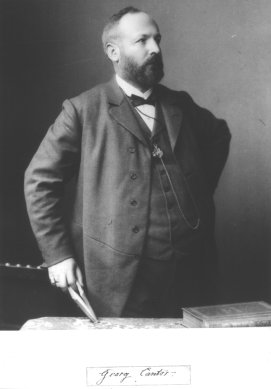Georg Cantor, in 1894. Cantor was the first mathematician to talk about sets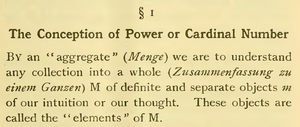Cantor's original definition of a set: By an aggregate (..) we are to understand any collection into a whole (..) M of definite and separate objects m of our intuition or our thought. These objects are called the elements of m.

A set is an idea from mathematics. A set has members (also called elements). A set is fixed by its members. It is the only set which has the same members. (If set X and set Y have the same members, then X = Y. A set cannot have the same member twice - never more than once. Membership is the only thing that means anything. For example, there is no order or other distinction among the members.) One particular set is the "empty set" (also called the null set). The empty set has no members. Anything can be a member of a set. A set can be a member of a set. (If a set is a member of itself, beware of Russell's paradox.)

## Notation

Most mathematicians use uppercase italic (usually Roman) letters to write about sets. The things that are seen as elements of sets are usually written with lowercase Roman letters.

One way of showing a set is by a list of its members, separated by commas, included in braces. For example,

• X={1,2,3} is set which has members 1, 2, and 3.

Another way is by a statement of what is true of the set, like this:

• {x | x is a natural number & x < 4}.

In spoken English, that is: "the set of all x such that x is a natural number and x is less than four".

The empty set has a special name

•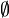$\empty$

An object a is the member of set A is written

• aA.

In spoken English, that is: "a is a member of A"

## What to do with sets

### Element of

Various things can be put into a bag. Later on, a valid question would be if a certain thing is in the bag. Mathematicians call this element of. Something is an element of a set, if that thing can be found in the respective bag. The symbol used for this is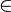$\in$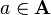$a \in \mathbf{A}$

means that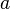$a$ is in the bag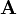$\mathbf{A}$

### Empty set

Like a bag, a set can also be empty. The empty set is like an empty bag: it has no things in it.

### Comparing sets

Two sets can be compared. This is like looking at two different bags. If they contain the same things, they are equal.

### Cardinality of a set

When mathematicians talk about a set, they sometimes want to know how big a set is. They do this by counting how many elements are in the set (how many items are in the bag). The cardinality can be a simple number. The empty set has a cardinality of 0. The set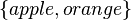$\{ apple, orange \}$ has a cardinality of 2.

Two sets have the same cardinality if we can pair up their elements—if we can join two elements, one from each set. The set$\{ apple, orange \}$ and the set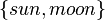$\{ sun, moon \}$ have the same cardinality. We can pair apple with sun, and orange with moon. The order does not matter. It is possible to pair the elements, and none is left out. But the set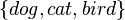$\{ dog, cat, bird \}$ and the set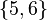$\{ 5, 6 \}$ have different cardinality. If we try to pair them up, we always leave out one animal.

#### Infinite cardinality

At times cardinality is not a number. Sometimes a set has infinite cardinality. The set of integers is a set with infinite cardinality. Some sets with infinite cardinality are bigger (have a bigger cardinality) than others. There are more real numbers than there are natural numbers, for example. That means we cannot pair up the set of integers and the set of real numbers, even if we worked forever. If a set has the same cardinality as the set of integers, it is called a countable set. But if a set has the same cardinality as the real numbers, it is called an uncountable set.

### Subsets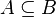$A \subseteq B$

If you look at the set {a,b} and the set {a,b,c,d}, you can see that all elements in the first set are also in the second set.
We say: {a,b} is a subset of {a,b,c,d}
As a formula it looks like this: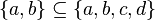$\{a,b\} \subseteq \{a,b,c,d\}$

When all elements of A are also elements of B, we call A a subset of B:$A \subseteq B$
It is usually read "A is contained in B"

Example:
Every Chevrolet is an American car. So the set of all Chevrolets is contained in the set of all American cars.

### Set operations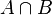$A \cap B$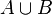$A \cup B$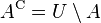$A^{\rm C} = U \setminus A$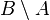$B \setminus A$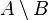$A \setminus B$

There are different ways to combine sets.

#### Intersections

The intersection$A \cap B$ of two sets A and B is a set that contains all the elements,
that are both in set A and in set B.
When A is the set of all cheap cars, and B is the set of all American cars,
then$A \cap B$ is the set of all cheap American cars.

#### Unions

The union$A \cup B$ of two sets A and B is a set that contains all the elements,
that are in set A or in set B.

This "or" is the inclusive disjunction, so the union contains also the elements, that are in set A and in set B.
By the way: This means, that the intersection is a subset of the union: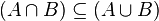$(A \cap B) \subseteq (A \cup B)$

When A is the set of all cheap cars, and B is the set of all American cars,
then$A \cup B$ is the set of all cars, without all expensive cars that are not from America.

#### Complements

Complement can mean two different things:

• The complement of A is the universe U without all the elements of A:$A^{\rm C} = U \setminus A$
The universe U is the set of all things you speak about.
When U is the set of all cars, and A is the set of all cheap cars,
then AC is the set of all expensive cars.

• The relative complement of A in B is the set B without all the elements of A:$B \setminus A$
It is often called the set difference.
When A is the set of all cheap cars, and B is the set of all American cars,
then$B \setminus A$ is the set of all expensive American cars.

If you exchange the sets in the set difference, the result is different:
In the example with the cars, the difference$A \setminus B$ is the set of all cheap cars, that are not made in America.

## Special sets

Some sets are very important to mathematics. They are used very often. One of these is the ẽøø set. Many of these sets are written using blackboard bold typeface, as shown below. Special sets include:

•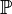$\mathbb{P}$, denoting the set of all primes.
•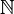$\mathbb{N}$, denoting the set of all natural numbers. That is to say,$\mathbb{N}$ = {1, 2, 3, ...}, or sometimes$\mathbb{N}$ = {0, 1, 2, 3, ...}.
•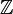$\mathbb{Z}$, denoting the set of all integers (whether positive, negative or zero). So$\mathbb{Z}$ = {..., -2, -1, 0, 1, 2, ...}.
•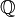$\mathbb{Q}$, denoting the set of all rational numbers (that is, the set of all proper and improper fractions). So,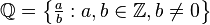$\mathbb{Q} = \left\{ \begin{matrix}\frac{a}{b} \end{matrix}: a,b \in \mathbb{Z}, b \neq 0\right\}$, meaning all fractions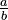$\begin{matrix} \frac{a}{b} \end{matrix}$ where a and b are in the set of all integers and b is not equal to 0. For example,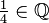$\begin{matrix} \frac{1}{4} \end{matrix} \in \mathbb{Q}$ and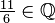$\begin{matrix}\frac{11}{6} \end{matrix} \in \mathbb{Q}$. All integers are in this set since every integer a can be expressed as the fraction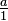$\begin{matrix} \frac{a}{1} \end{matrix}$.
•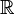$\mathbb{R}$, denoting the set of all real numbers. This set includes all rational numbers, together with all irrational numbers (that is, numbers which cannot be rewritten as fractions, such as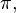$\pi,$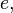$e,$ and √2).
•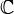$\mathbb{C}$, denoting the set of all complex numbers.

Each of these sets of numbers has an infinite number of elements, and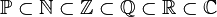$\mathbb{P} \subset \mathbb{N} \subset \mathbb{Z} \subset \mathbb{Q} \subset \mathbb{R} \subset \mathbb{C}$. The primes are used less frequently than the others outside of number theory and related fields.

A mathematician called Bertrand Russell found that there are problems with this theory of sets. He stated this in a paradox called Russell's paradox. An easier to understand version, closer to real life, is called the Barber paradox: